# Distributions for fitted lines

You can add a fitted line for the following distributions.

## Exponential distribution

Use the exponential distribution to model the time between events in a continuous Poisson process. It is assumed that independent events occur at a constant rate.

This distribution has a wide range of applications, including reliability analysis of products and systems, queuing theory, and Markov chains.

For example, the exponential distribution can be used to model:
• How long it takes for electronic components to fail
• The time interval between customers' arrivals at a terminal
• Service time for customers waiting in line
• The time until default on a payment (credit risk modeling)
• Time until a radioactive nucleus decays
The 2-parameter exponential distribution is defined by its scale and threshold parameters. The threshold parameter, θ, if positive, shifts the distribution by a distance θ to the right. For example, you are interested in studying the failure of a system with θ = 5. This means that the failures start to occur only after 5 hours of operation and cannot occur before. In the following graph, the threshold parameter, θ, is equal to 5, and shifts the distribution 5 units to the right.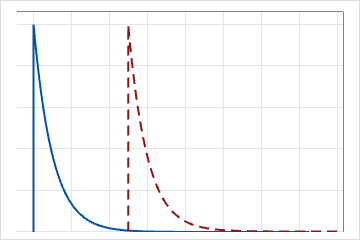For the 1-parameter exponential distribution, the threshold is zero, and the distribution is defined by its scale parameter. For the 1-parameter exponential distribution, the scale parameter equals the mean.

### What does memoryless mean?

An important property of the exponential distribution is that it is memoryless. The chance of an event does not depend on past trials. Therefore, the occurrence rate remains constant.

The memoryless property indicates that the remaining life of a component is independent of its current age. For example, random trials of a coin toss demonstrate the memoryless property. A system that has wear and tear, and thus becomes more likely to fail later in its life, is not memoryless.

## Gamma distribution

Use the gamma distribution to model positive data values that are skewed to the right and greater than 0. The gamma distribution is commonly used in reliability survival studies. For example, the gamma distribution can describe the time for an electrical component to fail. Most electrical components of a particular type will fail around the same time, but a few will take a long time to fail.

The gamma distribution is a continuous distribution that is defined by its shape and scale parameters. The 3-parameter gamma distribution is defined by its shape, scale, and threshold parameters. For example, in the following graph, the gamma distribution is defined by different shape and scale values when the threshold is set at 0.0. Notice that most values in a gamma distribution occur near each other, but some values trail into the upper tail.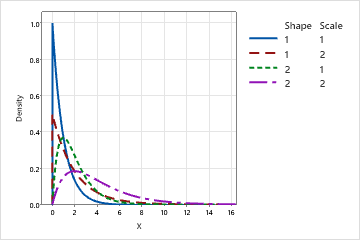When the shape parameter is an integer, the gamma distribution is sometimes called an Erlang distribution. The Erlang distribution is commonly used in queuing theory applications.

## Logistic distribution

Use the logistic distribution to model data distributions that have longer tails and higher kurtosis than the normal distribution.

The logistic distribution is a continuous distribution that is defined by its scale and location parameters. The logistic distribution has no shape parameter, which means that the probability density function has only one shape. The shape of the logistic distribution is similar to that of the normal distribution. However, the logistic distribution has longer tails.
Effect of scale parameter
The following graph shows the effect of the different values of the scale parameter on the logistic distribution.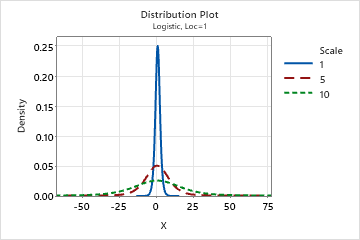Effect of location parameter
The following graph shows the effect of the different values of the location parameter on the logistic distribution.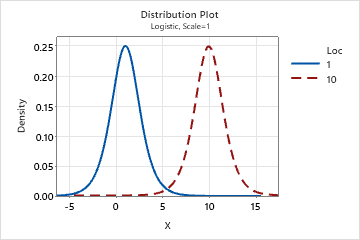## Loglogistic distribution

Use the loglogistic distribution when the logarithm of the variable is logistically distributed. For example, the loglogistic distribution is used in growth models and to model binary responses in fields such as biostatistics and economics.

The loglogistic distribution is a continuous distribution that is defined by its scale and location parameters. The 3-parameter loglogistic distribution is defined by its scale, location, and threshold parameters.

The following graph illustrates the loglogistic distribution for scale=1.0, location=0.0, and threshold=0.0.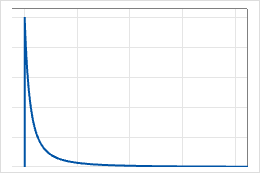The loglogistic distribution is also known as the Fisk distribution.

## Lognormal distribution

Use the lognormal distribution if the logarithm of the random variable is normally distributed. Use when random variables are greater than 0. For example, the lognormal distribution is used for reliability analysis and in financial applications, such as modeling stock behavior.

The lognormal distribution is a continuous distribution that is defined by its location and scale parameters. The 3-parameter lognormal distribution is defined by its location, scale, and threshold parameters.

The shape of the lognormal distribution is similar to that of the loglogistic and Weibull distributions. For example, the following graph illustrates the lognormal distribution for scale=1.0, location=0.0, and threshold=0.0.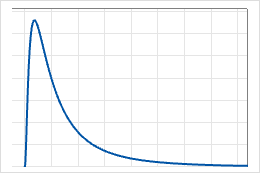## Normal distribution

The normal distribution is a continuous distribution that is specified by the mean (μ) and the standard deviation (σ). The mean is the peak or center of the bell-shaped curve. The standard deviation determines the spread of the distribution.

For example, in the following graph of a normal distribution, approximately, 68% of observations are within +/- 1 standard deviation of the mean; 95% are within +/- 2 standards deviations of the mean (as shown by the shaded area); and 99.7% are within +/- 3 standard deviations of the mean.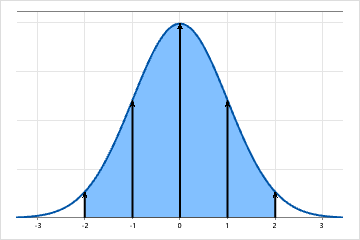The normal distribution is the most common statistical distribution because approximate normality occurs naturally in many physical, biological, and social measurement situations. Many statistical analyses assume that the data come from approximately normally distributed populations.

## Smallest and largest extreme value distributions

The largest extreme value distribution and the smallest extreme value distribution are closely related. For example, if X has a largest extreme value distribution, then −X has a smallest extreme value distribution, and vice versa.

### Smallest extreme value distribution

The smallest extreme value distribution is defined by its location and scale parameters. Use the smallest extreme value distribution to model the minimum value from a distribution of random observations. The smallest extreme value distribution is commonly used to model time to failure for a system that fails when its weakest component fails. The smallest extreme value distribution describes extreme phenomena such as the minimum temperature and rainfall during a drought. The smallest extreme value distribution is skewed to the left. For example, the distribution of the breaking strength of a chain is frequently skewed to the left, because the chain breaks when the weakest link breaks. This distribution has a few weak samples to the left and a majority of strengths in the upper tail.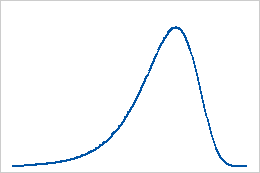### Largest extreme value distribution

The largest extreme value distribution is defined by its location and scale parameters. Use the largest extreme value distribution to model the maximum value from a distribution of random observations. The largest extreme value distribution describes extreme phenomena such as extreme wind velocities and high insurance losses. The largest extreme value distribution is skewed to the right. For example, the distribution of the water levels in a river over time is frequently skewed to the right with a few cases of extreme water levels to the right and a majority of water levels in the lower tail.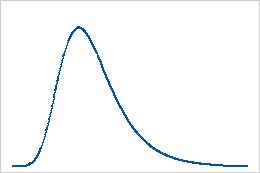## Weibull distribution

The Weibull distribution is a versatile distribution that can be used to model a wide range of applications in engineering, medical research, quality control, finance, and climatology. For example, the distribution is frequently used with reliability analyses to model time-to-failure data. The Weibull distribution is also used to model skewed process data in capability analysis.

The Weibull distribution is described by the shape, scale, and threshold parameters, and is also known as the 3-parameter Weibull distribution. The case when the threshold parameter is zero is called the 2-parameter Weibull distribution. The 2-parameter Weibull distribution is defined only for positive variables. A 3-parameter Weibull distribution can work with zeros and negative data, but all data for a 2-parameter Weibull distribution must be greater than zero.

Depending on the values of its parameters, the Weibull distribution can take various forms.

Effect of the shape parameter
The shape parameter describes how your data are distributed. A shape of 3 approximates a normal curve. A low value for shape, say 1, gives a right-skewed curve. A high value for shape, say 10, gives a left-skewed curve.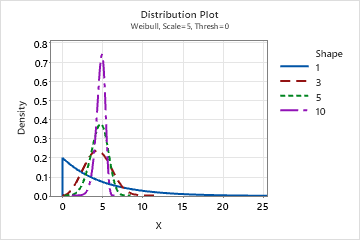Effect of the scale parameter
The scale, or characteristic life, is the 63.2 percentile of the data. The scale defines the position of the Weibull curve relative to the threshold, which is analogous to the way the mean defines the position of a normal curve. A scale of 20, for example, indicates that 63.2% of the equipment will fail in the first 20 hours after the threshold time.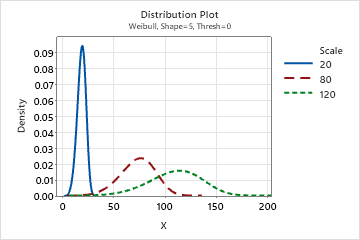Effect of the threshold parameter
The threshold parameter describes the shift of the distribution away from 0. A negative threshold shifts the distribution to the left, and a positive threshold shifts the distribution to the right. All data must be greater than the threshold. The 2-parameter Weibull distribution is the same as the 3-parameter Weibull with a threshold of 0. For example, the 3-parameter Weibull (3,100,50) has the same shape and spread as the 2-parameter Weibull (3,100), but is shifted 50 units to the right.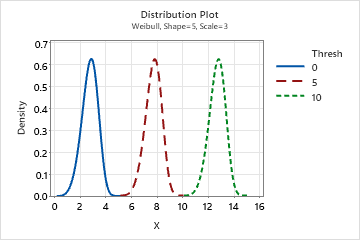By using this site you agree to the use of cookies for analytics and personalized content.  Read our policy